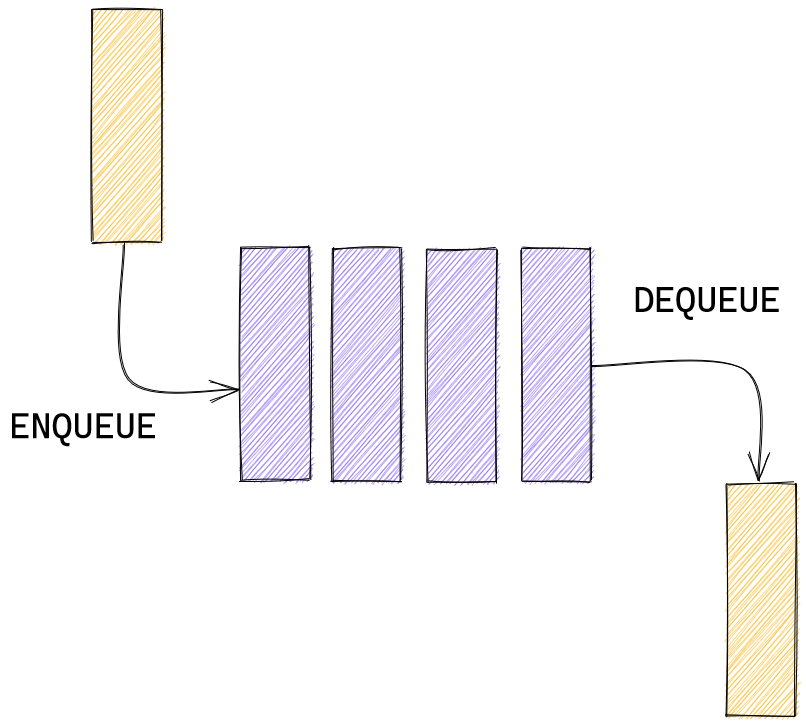# How to write a Queue in Rust

In this series I show the simplest way to build some data structures in Rust.

These implementations are not guaranteed to be the most performant. I only guarantee that they work as they are intended to work theoretically. If you are looking for the most performant solution consider using libraries (crates).

## What is a queue?

A queue is a very common data structure, which can be used in a variety of situations. It is needed for solving low level problems such as CPU job scheduling, but also for modeling a real-life queue - such as technical support requests that need to be processed in sequence. It is called Queue because it works exactly like a real queue: first in - first out (FIFO).

When we add something to a queue we say that we `enqueue` it. When we remove something from a queue we say that we `dequeue` it. Here's a simple model of how it works:Like a stack it can be implemented using `Vec`.

## Create

First of all, let us define a `struct` and call it `Queue`:

``````struct Queue<T> {
queue: Vec<T>,
}
``````

We want our queue to be able to store different kinds of types so we make it generic by using `T` instead of any specific type like `i32`.

Now, to create a new queue we can do this:

``````let q = Queue { queue: Vec::new() };
``````

But the more idiomatic way would be to define a new method for our queue, just like the one you used to create a new `Vec`:

``````impl<T> Queue<T> {
fn new() -> Self {
Queue { queue: Vec::new() }
}
}
``````

If you are not familiar with the `impl` (method) syntax you can read more about it here.

## Enqueue and dequeue

Both `enqueue` and `dequeue` are easy to implement. We can use methods that are available on `Vec`:

``````fn enqueue(&mut self, item: T) {
self.queue.push(item)
}

fn dequeue(&mut self) -> T {
self.queue.remove(0)
}
``````

Dequeuing can be done with `remove` - a very handy method which shifts (moves) the remaining elements to the left. The first item in the array we use to implement the queue is the head of our queue.

## Utility methods

There are some other methods that are associated with queues. They are `length`, `peek` and `is_empty`.

### length

Here we just reuse the `len` method of `Vec`.

``````fn length(&self) -> usize {
self.queue.len()
}
``````

### is_empty

Same for `is_empty`.

``````fn is_empty(&self) -> bool {
self.queue.is_empty()
}
``````

### peek

Here, again, we can use a very convenient method `first` that lets us take a look at the first item, or the head of our queue.

``````fn peek(&self) -> Option<&T> {
self.queue.first()
}
``````

Note that it returns a reference (`&`) and the reference itself is wrapped in an `Option`.

Now we have a functioning queue! Here's the full code:

``````struct Queue<T> {
queue: Vec<T>,
}

impl<T> Queue<T> {
fn new() -> Self {
Queue { queue: Vec::new() }
}

fn length(&self) -> usize {
self.queue.len()
}

fn enqueue(&mut self, item: T) {
self.queue.push(item)
}

fn dequeue(&mut self) -> T {
self.queue.remove(0)
}
fn is_empty(&self) -> bool {
self.queue.is_empty()
}

fn peek(&self) -> Option<&T> {
self.queue.first()
}
}
``````

And this is how you would you use it in code:

``````let mut queue: Queue<isize> = Queue::new();
queue.enqueue(1);
let item = queue.dequeue();
assert_eq!(item, 1);
assert_eq!(queue.is_empty(), true);
``````

You can also see the full code on my Github.